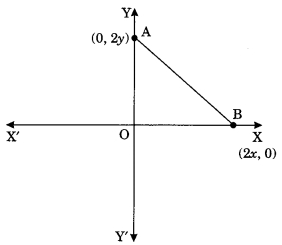# CBSEtips.in

## Thursday, 28 January 2021

### CBSE Class 10 Maths - MCQ Questions and Online Tests - Unit 7 - Coordinate Geometry

#### CBSE Class 10 Maths – MCQ and Online Tests – Unit 7 – Coordinate Geometry

Every year CBSE conducts board exams for 10th standard. These exams are very competitive to all the students. So our website provides online tests for all the 10th subjects. These tests are also very effective and useful for those who preparing for competitive exams like NEET, JEE, CA etc. It can boost their preparation level and confidence level by attempting these chapter wise online tests.

These online tests are based on latest CBSE Class 10 syllabus. While attempting these our students can identify the weak lessons and continuously practice those lessons for attaining high marks. It also helps to revise the NCERT textbooks thoroughly.

#### CBSE Class 10 Maths – MCQ and Online Tests – Unit 7 – Coordinate Geometry

Question 1.
The distance of the point P(2, 3) from the x-axis is
(a) 2
(b) 3
(c) 1
(d) 5

Question 2.
The distance between the points A(0, 6) and B(0, -2) is
(a) 6
(b) 8
(c) 4
(d) 2

Question 3.
The distance of the point P(-6, 8) from the origin is
(a) 8
(b) 2√7
(c) 10
(d) 6

Question 4.
The distance between the points (0, 5) and (-5, 0) is
(a) 5
(b) 5√2
(c) 2√5
(d) 10

Question 5.
AOBC is a rectangle whose three vertices are A(0, 3), O(0, 0) and B(5, 0). The length of its diagonal is
(a) 5
(b) 3
(c) $$\sqrt{34}$$
(d) 4

Answer: (c) $$\sqrt{34}$$

Question 6.
The perimeter of a triangle with vertices (0, 4), (0, 0) and (3, 0) is
(a) 5
(b) 12
(c) 11
(d) 7 + √5

Question 7.
The area of a triangle with vertices A(3, 0), B(7, 0) and C(8, 4) is
(a) 14
(b) 28
(c) 8
(d) 6

Question 8.
The points (-4, 0), (4, 0), (0, 3) are the vertices of a
(а) Right triangle
(b) Isosceles triangle
(c) Equilateral triangle
(d) Scalene triangle

Question 9.
The point which divides the lines segment joining the points (7, -6) and (3, 4) in ratio 1 : 2 internally lies in the

Question 10.
The point which lies on the perpendicular bisector of the line segment joining the points A(-2, -5) and B(2, 5) is
(a) (0, 0)
(b) (0, 2)
(c) (2, 0)
(d) (-2, 0)

Question 11.
The fourth vertex D of a parallelogram ABCD whose three vertices are A(-2, 3), B(6, 7) and C(8, 3) is
(a) (0, 1)
(b) (0, -1)
(c) (-1, 0)
(d)(1, 0)

Question 12.
If the point P(2, 1) lies on the line segment joining points A(4, 2) and B(8, 4), then
(a) AP = $$\frac{1}{3}$$ AB
(b) AP = PB
(c) PB = $$\frac{1}{3}$$ AB
(d) AP = $$\frac{1}{2}$$ AB

Answer: (d) AP = $$\frac{1}{2}$$ AB

Question 13.
If P ($$\frac{α}{3}$$, 4) is the mid-point of the line segment joining the points Q(-6, 5) and R(-2, 3), then the value of‘a’ is
(a) -4
(b) -12
(c) 12
(d) -6

Question 14.
The perpendicular bisector of the line segment joining the points A(l, 5) and B(4, 6) cuts the y-axis at
(a) (0, 13)
(b) (0, -13)
(c) (0, 12)
(d) (13, 0)

Question 15.
The coordinates of the point which is equidistant from the three vertices of the ΔAOB as shown in the figure.(a) (x, y)
(b) (y, x)
(c) ($$\frac{x}{2}$$, $$\frac{y}{2}$$)
(d) ($$\frac{y}{2}$$, $$\frac{x}{2}$$)

Question 16.
A circle drawn with origin as the centre passes through ($$\frac{13}{2}$$, 0). The point which does not lie in the interior of the circle is
(a) (-$$\frac{3}{4}$$, 1)
(b) (2, $$\frac{7}{3}$$)
(c) (5, –$$\frac{1}{2}$$)
(d) (-6, $$\frac{5}{2}$$)

Answer: (d) (-6, $$\frac{5}{2}$$)

Question 17.
A line intersects the y-axis and x-axis at the points P and Q respectively. If (2, -5) is the mid-point of PQ, then the coordinates of P and Q are respectively
(a) (0, -5) and (2, 0)
(b) (0, 10) and (-4, 0)
(c) (0, 4) and (-10, 0)
(d) (0, -10) and (4, 0)

Answer: (d) (0, -10) and (4, 0)

Question 18.
The area of a triangle with vertices (a, b + c), (b, c + a) and (c, a + b) is
(a) (a + b + c)²
(b) 0
(c) a + b + c
(d) abc

Question 19.
If the distance between the points (4, P) and (1, 0) is 5, then the value of P is
(a) 4 only
(b) ± 4
(c) -4 only
(d) 0

Question 20.
If the points A(1, 2), O(0, 0), C(a, b) are collinear, then
(a) a = b
(b) a = 2b
(c) 2a = b
(d) a = -b

Share: